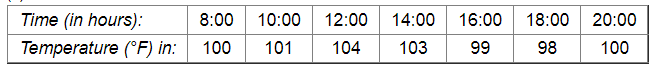# Draw the temperature-time graph in each of the following cases:

Question:

Draw the temperature-time graph in each of the following cases:

(i)

 Time (in hours): 7:00 9:00 11:00 13:00 15:00 17:00 19:00 21:00 Temperature (°F) in: 100 101 104 102 100 99 100 98

(ii)Solution:

Here, time is an independent variable and temperature is a dependent variable. So, we take time on the x-axis and temperature on the y-axis.

Let us choose the following scale:

For point (i):

On x-axis: 1 cm = 1 hours

On y-axis: 1 cm = 2οF

For point (ii):

On x-axis: 2 cm = 2 hours

On y-axis: 1 cm = 1οF

Let us assume that on the x-axis, the coordinate of origin is 6:00.

On y-axis, the coordinate of origin is 94οF .

So, the coordinates of O are (6:00, 94).

Now, let us plot (7:00, 100), (9:00, 101), (11:00, 104),..(21:00, 98) for point (i) and (8:00, 100), (10:00, 101), (12:00, 104),...,(20:00, 100) for point (ii). These points are joined to get the graphs representing the given information as shown in the figures below.​

(i)(ii)